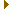# mightymandel v16

## GPU-based Mandelbrot set explorer

atom.c File Reference

### (v16)

Mandelbrot set atom finding. More...

#include <mpfr.h>
#include "atom.h"Include dependency graph for atom.c:

Go to the source code of this file.

## Macros

#define VARS   nx, ny, bx, by, wx, wy, zz, Ax, Ay, Bx, By, Cx, Cy, Dx, Dy, Ex, Ey, t1, t2, t3, t4, t5, t6, t7, t8, t9, t0, t01, t02, t03, t04

## Functions

int crosses_positive_real_axis (const mpfr_t ax, const mpfr_t ay, const mpfr_t bx, const mpfr_t by)
Check if a line segment intersects with the positive real axis.
int surrounds_origin (const mpfr_t ax, const mpfr_t ay, const mpfr_t bx, const mpfr_t by, const mpfr_t cx, const mpfr_t cy, const mpfr_t dx, const mpfr_t dy)
Check if a square surrounds the origin.
int did_escaped (const mpfr_t x, const mpfr_t y)
Check if a point escaped.
unsigned int boxperiod (const mpfr_t cx, const mpfr_t cy, const mpfr_t r, unsigned int maxperiod, void *abort_data, abort_t abort_fn)
Find the lowest period of any atom inside a box.
int muatom (int period, mpfr_t x, mpfr_t y, mpfr_t z, void *abort_data, abort_t abort_fn)
Find an atom of a given period.

## Detailed Description

Mandelbrot set atom finding.

Definition in file atom.c.

## Macro Definition Documentation

 #define VARS   nx, ny, bx, by, wx, wy, zz, Ax, Ay, Bx, By, Cx, Cy, Dx, Dy, Ex, Ey, t1, t2, t3, t4, t5, t6, t7, t8, t9, t0, t01, t02, t03, t04

Referenced by fpxx_approx_do(), and muatom().

## Function Documentation

 unsigned int boxperiod ( const mpfr_t cx, const mpfr_t cy, const mpfr_t r, unsigned int maxperiod, void * abort_data, abort_t abort_fn )

Find the lowest period of any atom inside a box.

Uses the Jordan curve method described here: http://mrob.com/pub/muency/period.html.

Parameters
 cx The real part of the box center. cy The imaginary part of the box center. r The box radius. maxperiod The maximum period to check until.
Returns
0 if no period was found, otherwise the found period.

Definition at line 87 of file atom.c.

References did_escaped(), and surrounds_origin().

Referenced by fpxx_start().Here is the call graph for this function:Here is the caller graph for this function:

 int crosses_positive_real_axis ( const mpfr_t ax, const mpfr_t ay, const mpfr_t bx, const mpfr_t by )

Check if a line segment intersects with the positive real axis.

Parameters
 ax The x coordinate of the first point. ay The y coordinate of the first point. bx The x coordinate of the second point. by The y coordinate of the second point.
Returns
1 if $$(a,b)$$ intersects $$(0,+\infty)$$, 0 otherwise.

Definition at line 23 of file atom.c.

Referenced by surrounds_origin().Here is the caller graph for this function:

 int did_escaped ( const mpfr_t x, const mpfr_t y )

Check if a point escaped.

This is a cheap inaccurate check using exponents. Inf/NaN are assumed escaped.

Parameters
 x The real part of the point. y The imaginary part of the point.
Returns
1 if the point escaped, 0 otherwise.

Definition at line 71 of file atom.c.

Referenced by boxperiod().Here is the caller graph for this function:

 int muatom ( int period, mpfr_t x, mpfr_t y, mpfr_t z, void * abort_data, abort_t abort_fn )

Find an atom of a given period.

Uses Newton's method as described here: http://mathr.co.uk/mandelbrot/bonds.pdf. The method is iterated with increased precision until the nucleus and $$\frac{1}{2}$$ bond are distint, which gives a size estimate and ensures the nucleus has sufficient precision.

Parameters
 period The period of the nucleus. x The real part of the nucleus (input: guess, output: nucleus). y The imaginary part of the nucleus (input: guess, output: nucleus). z The size of the nucleus (output valid when 1 is returned)
Returns
1 if the nucleus was accurately determined, 0 otherwise.

Definition at line 151 of file atom.c.

References VARS.

Referenced by fpxx_start().Here is the caller graph for this function:

 int surrounds_origin ( const mpfr_t ax, const mpfr_t ay, const mpfr_t bx, const mpfr_t by, const mpfr_t cx, const mpfr_t cy, const mpfr_t dx, const mpfr_t dy )

Check if a square surrounds the origin.

Parameters
 ax The x coordinate of the first point. ay The y coordinate of the first point. bx The x coordinate of the second point. by The y coordinate of the second point. cx The x coordinate of the third point. cy The y coordinate of the third point. dx The x coordinate of the fourth point. dy The y coordinate of the fourth point.
Returns
1 if $$(a,b)(b,c)(c,d)(d,a)$$ surround $$0$$, 0 otherwise.

Definition at line 54 of file atom.c.

References crosses_positive_real_axis().

Referenced by boxperiod().Here is the call graph for this function:Here is the caller graph for this function: